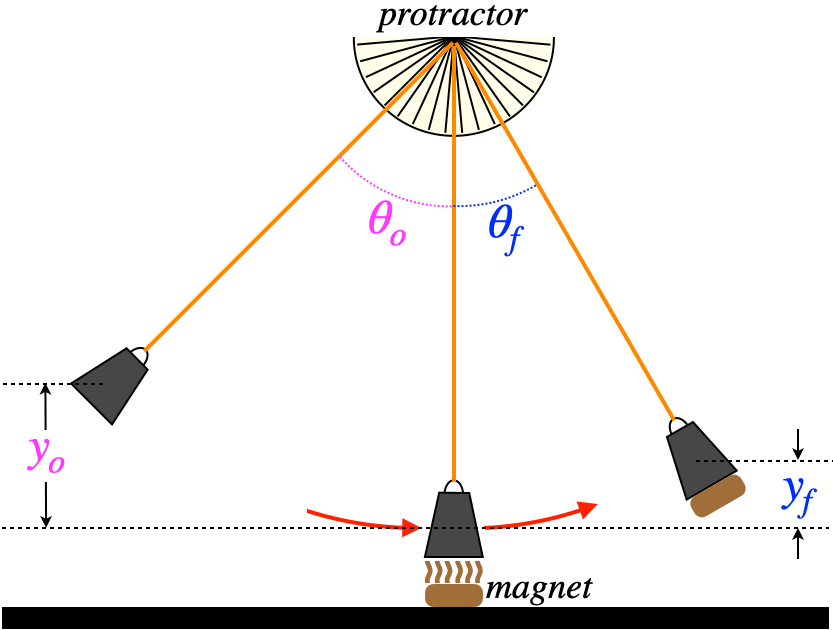# 7.1: Background Material

$$\newcommand{\vecs}{\overset { \rightharpoonup} {\mathbf{#1}} }$$ $$\newcommand{\vecd}{\overset{-\!-\!\rightharpoonup}{\vphantom{a}\smash {#1}}}$$$$\newcommand{\id}{\mathrm{id}}$$ $$\newcommand{\Span}{\mathrm{span}}$$ $$\newcommand{\kernel}{\mathrm{null}\,}$$ $$\newcommand{\range}{\mathrm{range}\,}$$ $$\newcommand{\RealPart}{\mathrm{Re}}$$ $$\newcommand{\ImaginaryPart}{\mathrm{Im}}$$ $$\newcommand{\Argument}{\mathrm{Arg}}$$ $$\newcommand{\norm}{\| #1 \|}$$ $$\newcommand{\inner}{\langle #1, #2 \rangle}$$ $$\newcommand{\Span}{\mathrm{span}}$$ $$\newcommand{\id}{\mathrm{id}}$$ $$\newcommand{\Span}{\mathrm{span}}$$ $$\newcommand{\kernel}{\mathrm{null}\,}$$ $$\newcommand{\range}{\mathrm{range}\,}$$ $$\newcommand{\RealPart}{\mathrm{Re}}$$ $$\newcommand{\ImaginaryPart}{\mathrm{Im}}$$ $$\newcommand{\Argument}{\mathrm{Arg}}$$ $$\newcommand{\norm}{\| #1 \|}$$ $$\newcommand{\inner}{\langle #1, #2 \rangle}$$ $$\newcommand{\Span}{\mathrm{span}}$$$$\newcommand{\AA}{\unicode[.8,0]{x212B}}$$

## Tarzan & Jane

The old legend of Tarzan of the Apes evokes the classic scene where Tarzan swings down from the trees on a vine to the ground, where is grabs his girlfriend Jane, and together they swing up to another branch (perhaps he rescues her from a dangerous animal or something). There is quite a lot of physics in a process like this, and in the lab that follows, we will examine it. We don't have Tarzan & Jane action figures to simulate this process in lab, so instead we'll swing an iron weight that picks up a magnet from the tabletop.  The process looks something like this:

Figure 7.1.1 – The Physical ProcessWhile it is easier to tie energy conservation to the physical situation using the heights $$y_o$$ and $$y_f$$, it is easier to measure the angles $$\theta_o$$ and $$\theta_f$$ in the lab.  So as preparation for this lab, you will need to do the geometry so that you are able to translate between these two sets of coordinates.  Assume that you know the length of the pendulum (which you can obviously measure in the lab).

## Conservation Laws

The text reference on the ballistic pendulum is extremely helpful here.  You should not be just copying-down equations from that example (because this is a different case), but rather make a note of what points during this process each conservation law (either momentum or energy) can be used, and why only that conservation law could be used in that situation.  These general principles will guide you for this case as well.

## Important Preparation

The starting and initial heights are clearly related to each other.  For example, when the initial height is greater, the final height is also greater.  When it comes to expressing the relationship between these heights mathematically, we have (as already mentioned) the conservation laws.  But the number of physical parameters from which this mathematical relationship is built (especially if friction is ignored) is two – the masses of the iron weight ($$M$$) and magnet ($$m$$).  It should therefore be clear what needs to be done in preparation for this lab, no matter what its ultimate goal might be: Determine the mathematical relationship between $$y_o$$, $$y_f$$, $$M$$, and $$m$$.

This page titled 7.1: Background Material is shared under a CC BY-SA 4.0 license and was authored, remixed, and/or curated by Tom Weideman directly on the LibreTexts platform.